Linear Regression

Example Codes:

Linear regression is an approach of regression analysis that characterize the relationship between a continuous response and explanatory variables of interest. Simple linear regression model contains only a single explanatory variable, while multiple linear regression contains more than one predictor.

Linear regression model describes the linear relationship between the response variable and predictors, where the parameters are estimated from the data. There are various methods to fit the linear regression model, among which the least square technique is most common.

# Assumptions

There are four assumptions of the linear regression model:

-        Linearity: The mean of the response variable has linear relationship with the predictors.

-        Homoscedasticity: The variance of errors is the same across each value of the predictors.

-        Independence: The errors are independent.

-        Normality: The errors follow normal distribution with zero mean.

# Formula and interpretation

The formula of a linear regression model has the following form: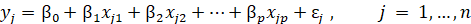, where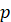is the number of predictors,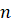is the number of observations.

Predicted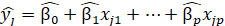For a fitted linear regression model, the value of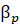is the expected change in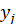for one-unit change in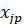.

# Example Code in SAS

TITLE1 " COMPARING SAME MEANS USING GLM PROCEDURE " ;

DATA STUDY ; INPUT COLOUR \$ NAME \$ ID RTIME ;

DATALINES ;

GREEN ABEL 1 232.6

RED ABEL 1 232.0

GREEN AMOS 3 253.1

RED AMOS 3 237.1

GREEN ANDY 4 205.4

RED ANDY 4 201.5

GREEN BART 5 226.0

RED BART 5 211.1

RUN; ** NOTE: MOST DATASETS HAVE A LINE OF DATA FOR EACH SUBJECT;

/* Simple linear regression */

TITLE1 " ASSUMING A COMPLETELY RANDOMIZED DESIGN " ;

PROC GLM DATA = STUDY ; CLASS COLOUR ;

MODEL RTIME = COLOUR ;

LSMEANS COLOUR / TDIFF PDIFF STDERR CL ; RUN ;

/* Multiple linear regression */

TITLE1 " ASSUMING A RANDOMIZED BLOCK DESIGN " ;

PROC GLM DATA = STUDY ; CLASS COLOUR ID ;

MODEL RTIME = COLOUR ID ; **Note ID in MODEL statement ;

LSMEANS COLOUR / TDIFF PDIFF STDERR CL ; RUN ;

# Example Code in R

# input data

colour <- c("green", "red", "green", "red", "green", "red", "green", "red",

"green", "red")

"bart", "bart")

id <- c(1, 1, 2, 2, 3, 3, 4, 4, 5, 5)

rtime <- c(232.6, 232, 257.5, 250.5, 253.1, 237.1, 205.4, 201.5, 226, 211.1)

# identify colour, name and id as categorical variables

colour <- factor(colour)

name <- factor(name)

id <- factor(id)

# create the dataframe

df <- data.frame(colour, name, id, rtime)

# Simple linear regression

glm(formula = rtime ~ colour, data = df)

# Multiple linear regression

glm(formula = rtime ~ colour + id, data = df)